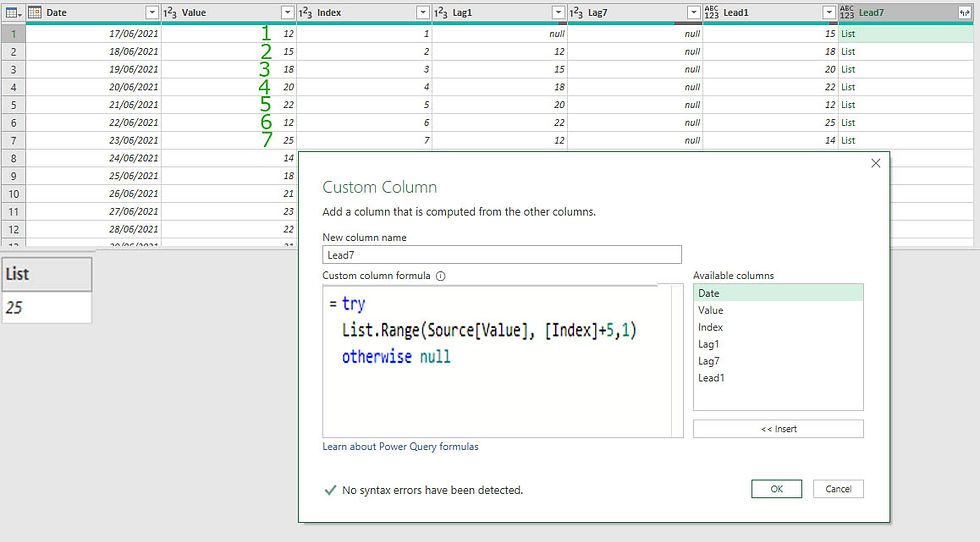top of page
Search
• Marek Vavrovic

# Calculate Lag and Lead value using power query.

Lag and Lead are functions used in many programming languages that provides access to a row at a specified physical offset which comes before (Lag) or after (Lead) the current row. These functions can be very useful for calculating the difference between the current row and the previous row or the next row.

Method 1

Data source:

Load data into Power Query and add an Index starting at 1 incrementing by 1.Step 1: Calculating Lag (1) using List.Range function and an Index.

Syntax:

`List.Range(list as list, offset as number, optional count as nullable number) as list `

Returns a subset of the list beginning at the offset list. An optional parameter, offset, sets the maximum number of items in the subset.

Lag(1)=List.Range(Source[Value], [Index]-2,1)The first row returned an error, to get rid of it add to the code: if [Index]=1 then null else List.Range(Source[Value], [Index]-2,1))

or use try [code] otherwise nullStep 2: Click on the double arrow of the Lag1 column > Expand to new rows.

This will generate the previous row value. If you need to calculate the Net Change simply add a Custom column and the formula will be: [Value]-[Lag1]To access a row at a offset (-7) use:

try

List.Range(Source[Value], [Index]-8,1)

otherwise null

(Power Query is based zero.)Step 3: Lead(1) This returns the value from the next row in order and the null value will be on the bottom.

List.Range(Source[Value], [Index],1)

otherwise nullStep 4: Lead(7) If we need to return the 7th row.

List.Range(Source[Value], [Index]+5,1)

otherwise nullMethod 2

Lag(1)

Step 1: Add a Custom Index starting at -1 , increment 1Step 2: Add a Custom column referencing the last step from the applied step panel. This will return whole table for each row.Step 3: using positional operator { } and lookup column operator [ ]

Using column Index as a positional operator returns previous record for every single row.You can specify which column from the record you want to return by using the field access operator.To calculate the Net change:

NetChange= try

otherwise null8,828 views1 comment

See All
bottom of page